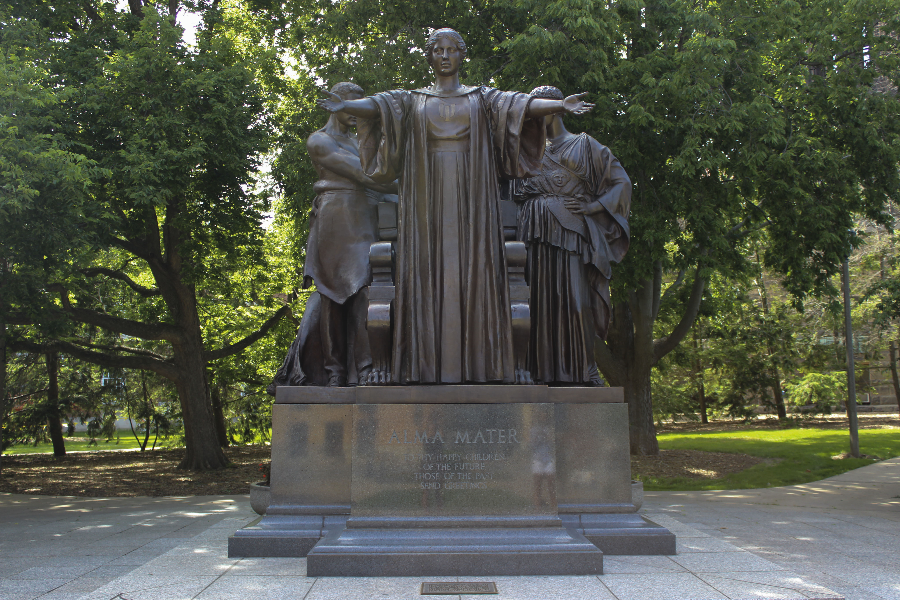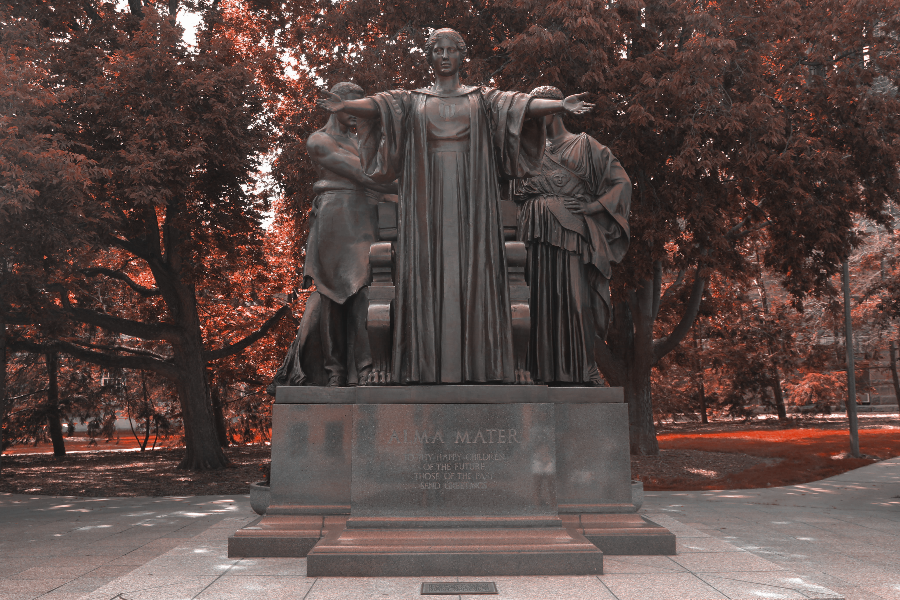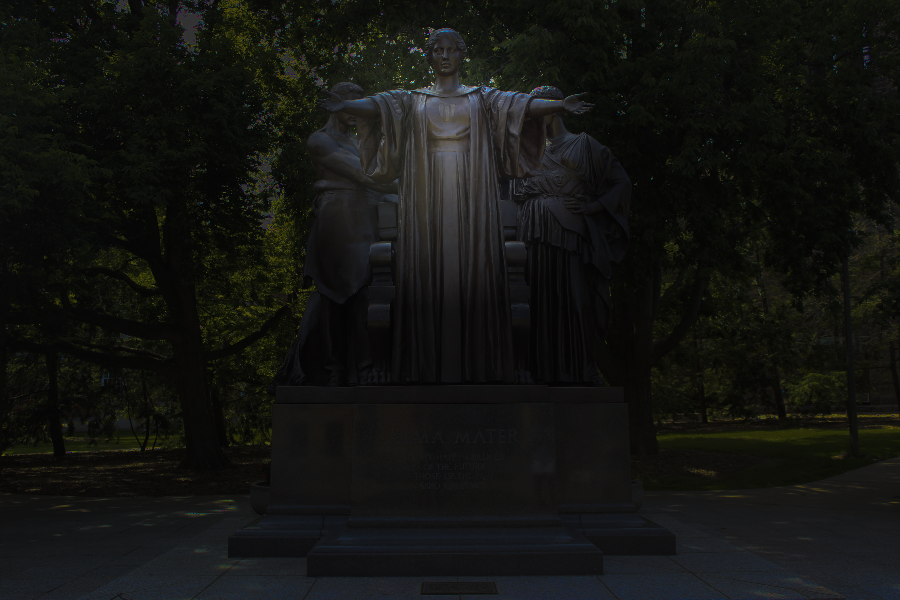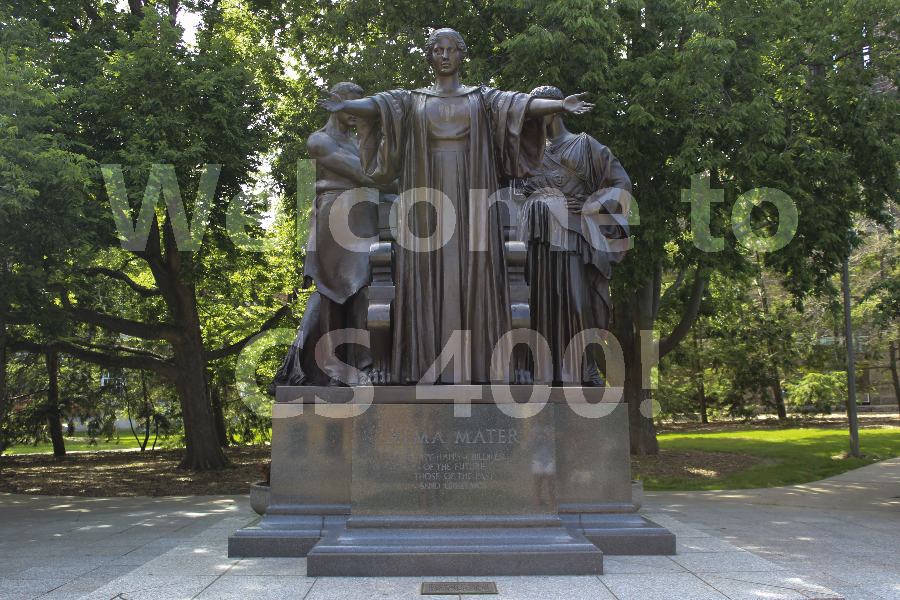# Image Transformations in C++

Published:

## Introduction

This project was a part of the final week assignment of an online course on Coursera on Object-Oriented Data Structures in C++ taught by Prof. Ulmschneider from department of computer science at UIUC. It is such a well designed course for people from all background who are interested in understanding the essential components of a C++ code. The goal of this project was to transform the given image by changing the Hue, Saturation or Luminance of the pixel.

## Illinify

The first part of the assignment was to ‘Illinify’ the image, which is explained as follows. Hue itself refers to the color of the pixel. The color range is defined in between 0 and 360. The hue of each pixel should be set to either ‘Illini orange’ which has a hue of 11 or ‘Illini Blue’ which has a hue of 216, depending on which hue value the original hue of the pixel is closer to.PNG illinify(PNG image) {

for (unsigned x = 0; x < image.width(); x++) {
for (unsigned y = 0; y < image.height(); y++) {
HSLAPixel & pixel = image.getPixel(x, y);

// pixel is a reference to the memory stored inside of the PNG image,
// which means you're changing the image directly. No need to set
// the pixel since you're directly changing the memory of the image.
pixel.h=11;
if(pixel.h >= 113.5 && pixel.h <=293.5) pixel.h=216;
}
}
return image;
}


## Spotlight

The second part of the project was to put a spotlight on the image. What this means is to decrease the luminance (or brightness of the pixels) with increasing radial distance around a central pixel. In this case the luminance was decreased by 0.5% per pixel unit in euclidean distance with a maximum of 80% decrease.PNG createSpotlight(PNG image, int centerX, int centerY) {

for (unsigned x = 0; x < image.width(); x++) {
for (unsigned y = 0; y < image.height(); y++) {
HSLAPixel & pixel = image.getPixel(x, y);
double distance=sqrt((x-centerX)*(x-centerX)+(y-centerY)*(y-centerY));
double decrease_factor = 1-distance*0.5/100 ;
if (distance>160) decrease_factor=0.2;
// pixel is a reference to the memory stored inside of the PNG image,
// which means you're changing the image directly. No need to set
// the pixel since you're directly changing the memory of the image.
pixel.l = decrease_factor*pixel.l;
}
}
return image;

}


## Watermarking

The third part of the project required putting a watermark on the image. This requires the use of an overlay image as a stencil, to find out the pixels in the Alma image that intersects with overlay image where the luminance is 1. The luminance of such pixels in original image were increased by 0.2 (with a maximum allowed value upto 1).PNG watermark(PNG firstImage, PNG secondImage) {

for (unsigned x = 0; x < secondImage.width(); x++) {
for (unsigned y = 0; y < secondImage.height(); y++) {
HSLAPixel & pixel2 = secondImage.getPixel(x, y);
HSLAPixel & pixel1 = firstImage.getPixel(x,y);
//if(pixel2.l==1){pixel1.l+=0.2;}
if(pixel2.l==1){
if(pixel1.l+0.2<1){
pixel1.l+=0.2;
}
else{
pixel1.l=1;
}
}
// pixel is a reference to the memory stored inside of the PNG image,
// which means you're changing the image directly. No need to set
// the pixel since you're directly changing the memory of the image.
}
}
return firstImage;
}


Tags: